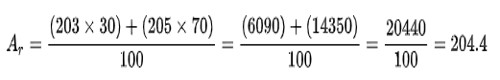A relative atomic mass (symbol: Ar) is a measure of how heavy atoms are. It is the ratio of the average mass of one atom of an element to one-twelfth of the mass of an atom of carbon-12. In other words, a relative atomic mass tells you the number of times an average atom of an element from a given sample is heavier than one-twelfth of an atom of carbon-12. For example, for the oxygen atom, its rest mass is 2.657×10-26, which divides it by 1.661×10-27 (2.657×10-26/1.661×10-27 ) and the answer will approximately be 16, that is the relative atomic mass for oxygen.

The number of protons an atom has determines what element it is. However, most elements in nature consist of atoms with different numbers of neutrons. An atom of an element with a certain number of neutrons is called an isotope. For example, the element thallium has two common isotopes: thallium-203 and thallium-205. Both isotopes of thallium have 81 protons, but thallium-205 has 124 neutrons, 2 more than thallium-203, which has 122. Each isotope has its own mass, called its isotopic mass.

We can find the relative atomic mass of a sample of an element by working out the abundance-weighted mean of the relative isotopic masses. For example, if a sample of thallium is made up of 30% thallium-203 and 70% thallium-205,A standard atomic weight is the mean value of relative atomic masses of a number of normal samples of the element. Standard atomic weight values are published at regular intervals by the Commission on Isotopic Abundances and Atomic Weights of the International Union of Pure and Applied Chemistry (IUPAC). The standard atomic weight for each element is on the periodic table.

Often, the term relative atomic mass is used to mean standard atomic weight. It is numerically equal to the relative atomic mass (or atomic weight) in grams. This is not quite correct, because relative atomic mass is a less specific term that refers to individual samples. For example, a sample from another planet could have a relative atomic mass very different from the standard Earth-based value.

Relative atomic mass is not the same as:

• atomic mass (symbol: ma), which is the mass of a single atom, commonly expressed in unified atomic mass units
• mass number (symbol: A), which is the sum of the number of protons and the number of neutrons in the nucleus of an atom
• atomic number (symbol: Z), which is the number of protons in the nucleus of an atom.